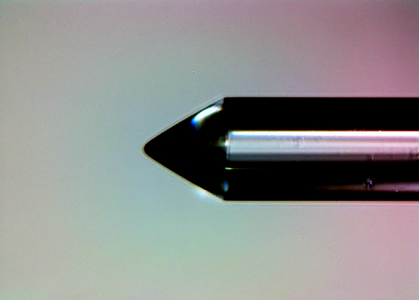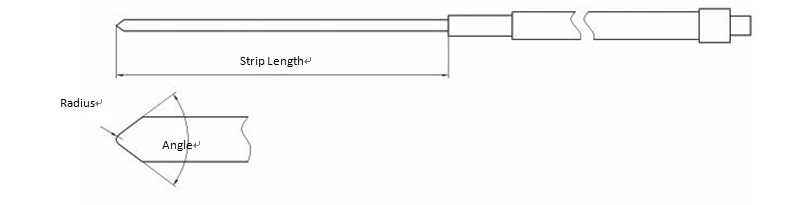### Current Position：Home / Products

 Tapered Lensed Optical FibersProduct Features Product Applications The tapered lensed optical fiber is first grinded into a tapered shape on the end face of the optical fiber by the precision grinding equipment, and then the optical microsphere lens is processed at its tip, so as to expand the numerical aperture of the optical fiber and increase the absorption capacity. Couplings of various type of laser device Spherical lens Low loss Excellent coupling efficiency High precision
 Specifications Parameter Unit Values Fiber Type / Conventional single-mode / multimode / polarization maintaining fiber, special optical fibers provided by customers Angle ° （70°~100°）±1° Radius of Curvature μm 7~8 μm±1 μm or radius specified by customers Strip Length mm Length specified by customers±1mm Anti-Reflection Coating / 780,830,840,980,1310,1480,1550nm Reflectance Max. 0.3%
 Package Size DiagramOrdering Information T S L F 0 Fiber Type 1=G652 or equivalent 5=980-20 or equivalent 6=HI060 or equivalent 9=HI780 S=Specify Angle 1=70°±1° 2=80°±1° 3=90°±1° 4=100°±1° S=Specify Radius 1=5μm±1μm 2=6μm±1μm 3=7μm±1μm 4=8μm±1μm 5=9μm±1μm S=Specify Strip Length 1=5mm 2=10mm 3=15mm 4=20mm S=Specify AR Coating 1=780nm 2=830nm 4=840nm 5=980nm 6=1310nm 7=1480nm 8=1550nm S=Specify Pigtail Type S=250um bare fiber M=900um loose tube Pigtail Length 0=0.5m 1=0.75m 2=1.0m 3=1.5m 4=2.0m S=specify Connector 0=None 1=FC/PC 2=FC/SPC 3=FC/APC 7=FC/UPC